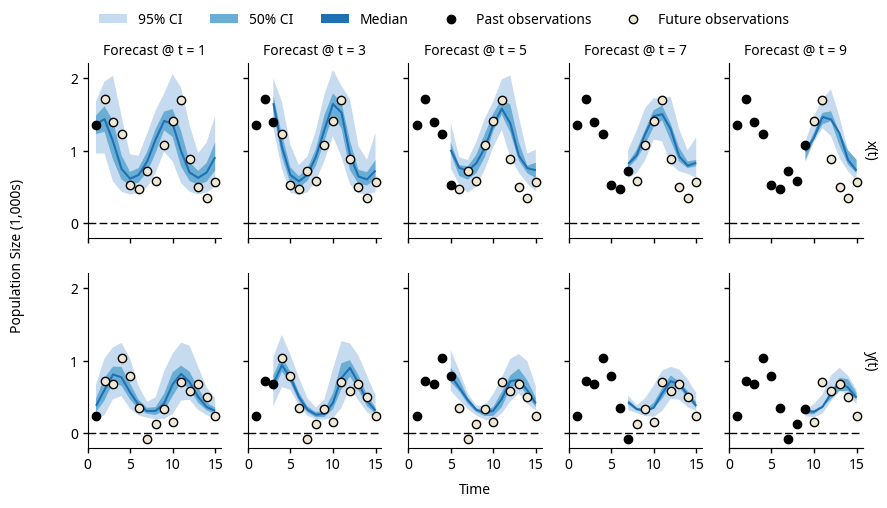# Bootstrap particle filter for Python¶

Welcome to the pypfilt documentation. This package implements a bootstrap particle filter that can be used for recursive Bayesian estimation and forecasting.

If there is a system or process that can be:

• Described (modelled) with mathematical equations; and
• Measured repeatedly in some (noisy) way.

Then you can use pypfilt to estimate the state (and parameters) of this system.

The Getting Started guide shows how to estimate the size of prey and predator species populations, and how to generate forecasts that predict the future sizes of these populations.Example forecasts of the prey population $$x(t)$$ and the predator population $$y(t)$$, generated at different times and using noisy observations of both populations.